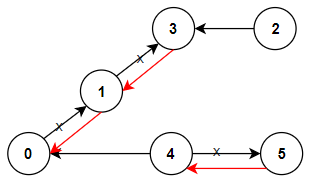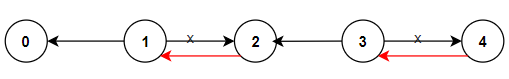@TOC

# # 题目描述

`n` 座城市，从 `0``n-1` 编号，其间共有 `n-1` 条路线。因此，要想在两座不同城市之间旅行只有唯一一条路线可供选择（路线网形成一颗树）。去年，交通运输部决定重新规划路线，以改变交通拥堵的状况。``````输入：n = 6, connections = [[0,1],[1,3],[2,3],[4,0],[4,5]]

````````````输入：n = 5, connections = [[1,0],[1,2],[3,2],[3,4]]

``````

``````输入：n = 3, connections = [[1,0],[2,0]]

``````

1. `2 <= n <= 5 * 10^4`
2. `connections.length == n-1`
3. `connections[i].length == 2`
4. `0 <= connections[i], connections[i] <= n-1`
5. `connections[i] != connections[i]`

# # 解题方法

## # DFS

Python 代码如下：

``````class Solution:
def minReorder(self, n: int, connections: List[List[int]]) -> int:
graph = collections.defaultdict(dict)
for con in connections:
graph[con][con] = 1
graph[con][con] = 0
visited = set()
return self.dfs(graph, 0, visited)

def dfs(self, graph, cur, visited):
res = 0
for nxt, value in graph[cur].items():
if nxt not in visited:
res += value
res += self.dfs(graph, nxt, visited)
return res
``````

## # BFS

``````class Solution:
def minReorder(self, n: int, connections: List[List[int]]) -> int:
graph = collections.defaultdict(dict)
for con in connections:
graph[con][con] = 1
graph[con][con] = 0
queue = collections.deque()
queue.append(0)
visited = set()
res = 0
while queue:
cur = queue.popleft()